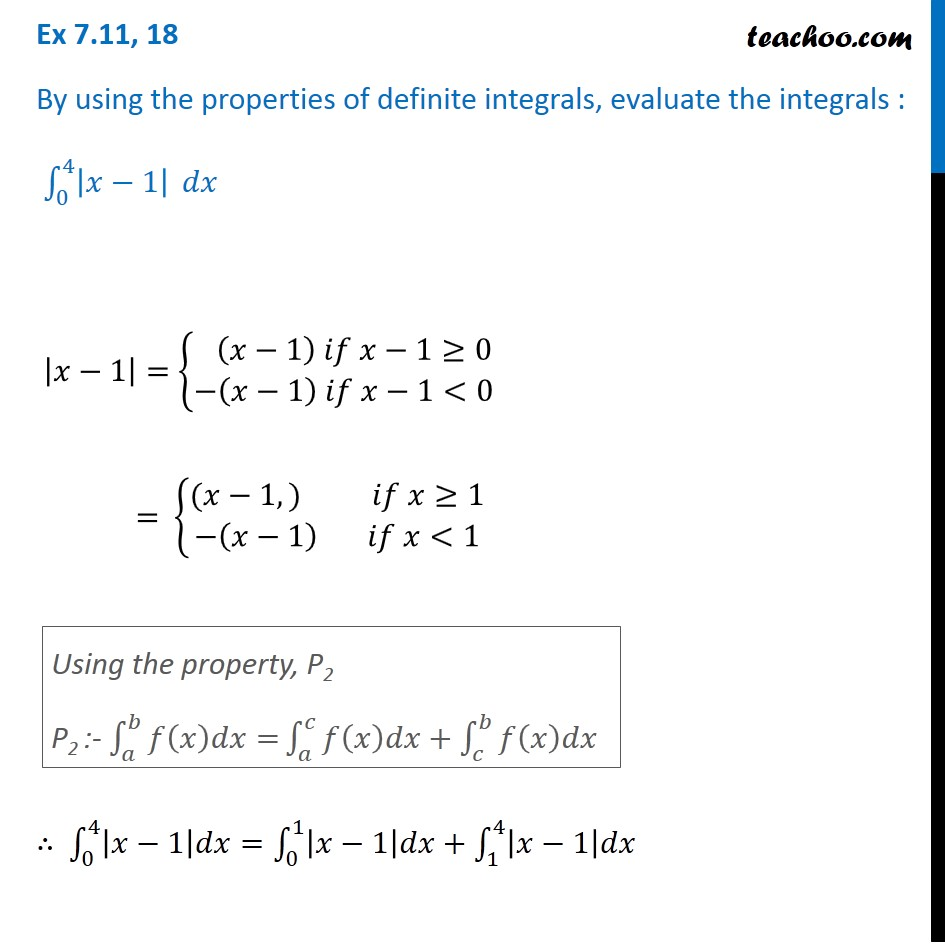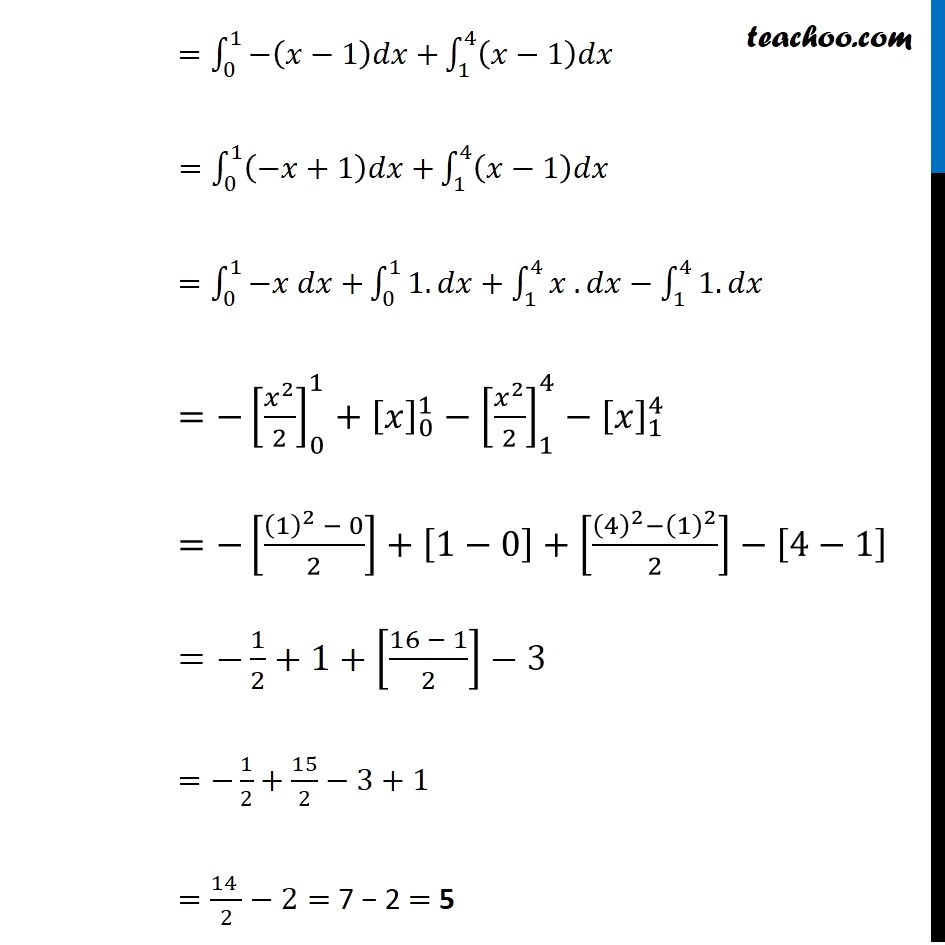1. Chapter 7 Class 12 Integrals (Term 2)
2. Serial order wise
3. Ex 7.11

Transcript

Ex 7.11, 18 By using the properties of definite integrals, evaluate the integrals : ∫_0^4▒|𝑥−1| 𝑑𝑥 |𝑥−1|= {█( (𝑥−1) 𝑖𝑓 𝑥−1≥0@−(𝑥−1) 𝑖𝑓 𝑥−1<0)┤ = {█((𝑥−1,) 𝑖𝑓 𝑥≥1@−(𝑥−1) 𝑖𝑓 𝑥<1)┤ ∴ ∫_0^4▒|𝑥−1|𝑑𝑥=∫_0^1▒|𝑥−1|𝑑𝑥+∫_1^4▒|𝑥−1|𝑑𝑥 Using the property, P2 P2 :- ∫_𝑎^𝑏▒〖𝑓(𝑥)𝑑𝑥=〗 ∫_𝑎^𝑐▒〖𝑓(𝑥)𝑑𝑥+∫_𝑐^𝑏▒𝑓(𝑥)𝑑𝑥〗 =∫_0^1▒〖−(𝑥−1)𝑑𝑥+〗 ∫_1^4▒(𝑥−1)𝑑𝑥 =∫_0^1▒〖(−𝑥+1)𝑑𝑥+〗 ∫_1^4▒(𝑥−1)𝑑𝑥 =∫_0^1▒〖−𝑥 𝑑𝑥+〗 ∫_0^1▒〖1. 𝑑𝑥+∫_1^4▒〖𝑥 . 𝑑𝑥−∫_1^4▒〖1.𝑑𝑥〗〗〗 =−[𝑥^2/2]_0^1+[𝑥]_0^1−[𝑥^2/2]_1^4−[𝑥]_1^4 =−[((1)^2 − 0)/2]+[1−0]+[((4)^2−(1)^2)/2]−[4−1] =−1/2+1+[(16 − 1)/2]−3 =−1/2+15/2−3+1 =(14 )/2−2= 7 − 2 = 5

Ex 7.11

Chapter 7 Class 12 Integrals (Term 2)
Serial order wise